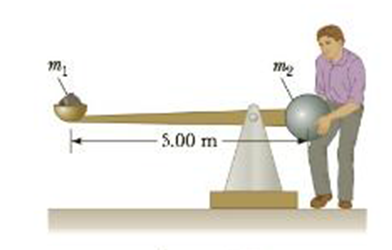Chapter 8, Problem 89AP

Chapter
Section
Textbook Problem

A war-wolf, or trebuchet, is a device used during the Middle Ages to throw rocks at castles and now sometimes used to fling pumpkins and pianos. A simple trebuchet is shown in Figure P8.89. Model it as a stiff rod of negligible mass 5.00 m long and joining particles of mass m1 = 0.120 kg and m2 = 60.0 kg at its ends. It can turn on a frictionless horizontal axle perpendicular to the rod and 14.0 cm from the particle of larger mass. The rod is released from rest in a horizontal orientation. Find the maximum speed dial the object of smaller mass attains.FigureP8.89

To determine
The magnitude of maximum speed of the smaller mass.

Explanation

Given Info:

Length of the stiff rod is 3.00m , mass of the small particle 0.120kg , mass of the big particle 60.0kg , the distance of the smaller particle from the axle is 2.86m , the distance of the larger particle from the axle is 0.14m .

Formula to calculate initial total mechanical energy is,

ET,i=PEi+KEi

• ET,i is the initial total mechanical energy of the system
• PEi is the initial potential energy of the system
• KEi is the initial kinetic energy of the system

Consider the reference plane as the horizontal rotational axis. The potential energy of the system is zero at the reference plane. Initially both kinetic and potential energy of the system will be zero

ET,i=0

Formula to calculate final total mechanical energy is,

ET,f=PEf+KEf

• ET,f is the final total mechanical energy of the system
• PEf is the final potential energy of the system
• KEf is the final kinetic energy of the system

Formula to calculate the final kinetic energy of the system is given by

KEf=12I1ω2max+12I2ω2max

• I1 is the moment of inertia of the small mass
• I2 is the moment of inertia of the big mass
• ωmax is the maximum  angular speed

The formula to calculate I1 is,

I1=m1r21

• m1 is the mass of small particle
• r1 is the distance of the small mass from the axle

The formula to calculate I2 is,

I2=m2r22

• m2 is the mass of big particle
• r2 is the distance of the big particle from the axle

The formula to calculate the total potential energy of the system is,

PEf=m1gr1m2gr2

• g is the acceleration due to gravity

Use 12I1ω2max+12I2ω2max for KEf and m1gr1m2gr2 for PEf in ET,f=PEf+KEf to rewrite ET,f .

ET,f=12(m1r21)ω2max+12(m2r22)ω2max+m1gr1m2gr2

According to conservation of mechanical energy of the system sum of both kinetic and potential energy of the system before and after releasing of the small particle will be same. The smaller particle will attain maximum velocity when the rod becomes vertical with respect to the ground.

ET,f=0

Substitute 0 for ET,f in the above equation and rewrite in terms of ωmax

Still sussing out bartleby?

Check out a sample textbook solution.

See a sample solution

The Solution to Your Study Problems

Bartleby provides explanations to thousands of textbook problems written by our experts, many with advanced degrees!

Get Started